## Forecasting the upcoming-elections-in-the-us-extracting-tweets

#### Introduction

Objective: Extraction of tweets and Perform sentiment analysis on the presidential candidature of Donald Trump, Joe Biden and Kanye West in the upcoming elections in US in November, 2020.

In this project we've extracted tweets using Twitter's API, and GetOldTweets library to overcome the timeframe limitations of Twitter API. After extraction, we have done preprocessing for cleaning the datasets, basic EDA, sentiment analysis to observe polarity towards each candidate, used classification models on these sentiments and created visualizations. And the end result was in favour of Joe Biden.We can predict that the people were most inclined towards Joe Bidden.

Preprocessing Of Tweets

Preprocessing of our data is very vital for this project as it pertains to cleaning actual tweets by users. This cleaning will prove to be a massive factor for the success rate of our project. This reduces complexity of data under analysis as data in real world is unclean. The preprocessing that was carried out in this project was mainly done on the tweets.

Firstly, the data has to be cleaned. For this we used various methods like checking for any null values , checking for duplicates ( & if any to remove them). After checking this, we move on with the actual preprocessing. There were many methods used. They are listed below –

• Using Regular Expressions to remove Emojis from Tweets

• Using Regular Expressions to remove any retweets (if they exist)

• Using Regular Expressions to remove the usernames from the tweets as they do not provide any additional information

• Using Regular Expressions to remove any URLs, websites,etc

• Using Regular Expressions to identify for any hashtags in the tweet, & if they exist, remove the hashtag & keep the word. This can be very useful when modelling as it does not remove any possible words that might be a major factor in calculating the sentiment

• Using Regular Expressions to remove any special characters, numbers , punctuations

• Converting everything to lower case

• Lastly, we used the Tweet-Preprocessor Module for cleaning any leftover junk.

This was the major portion of preprocessing our tweets. Next, we have to get it ready for calculating our sentiment as well. So we will be performing certain tasks using NLTK Module. These are listed below –

• NLTK Module was used to tokenize all words

• NLTK Module was used for removing any existing stop words (eg. Or , from , them, Does , etc)

• NLTK Module was used to perform stemming

• Words that were less than a length of 2 were dropped

Sentiment Analysis

Sentiment Analysis refers to processing and analyzing text and categorizing it into different states and emotions. Natural Language Processing is used for performing sentiment analysis, which is a subfield of artificial intelligence and linguistics to process and analyze large amounts of natural data.

To calculate the Sentiment of each tweet, we used the vader_lexicon file from NLTK which was used from the SentimentIntensityAnalyzer() package from NLTK module. Using this we calculated the polarity of each tweet & created a dataframe. Each tweet was classified as Negative, Neutral, Positive & Compound with a score between zero & one pertaining to how close the tweet is to the column. The final sentiment was decided on the final score of the compound column. ( If less than zero, it would be classified as negative; greater than zero, it would be classified as positive; zero then neutral) Using these , we proceeded with making the final model. Some Visual Analysis of our outcome:

### Joe Biden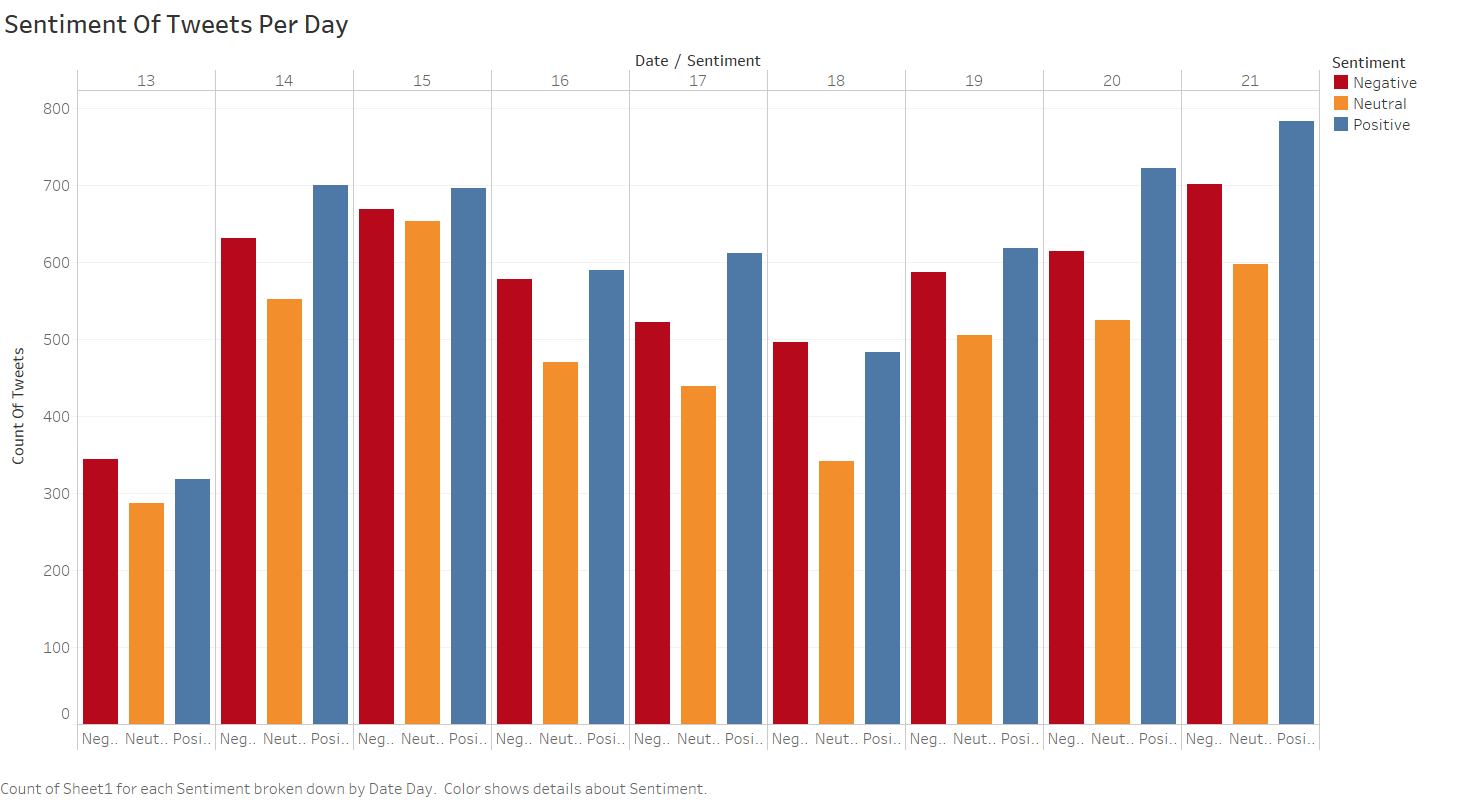##### In the above graph, we have the three broad sentiments on X axis and No. of tweets of that particular sentiment in our dataset, along with the dates of them being tweeted. A general observation here is that there is a majority of positive tweets on most of days. So, the general reaction towards Biden is affirmative.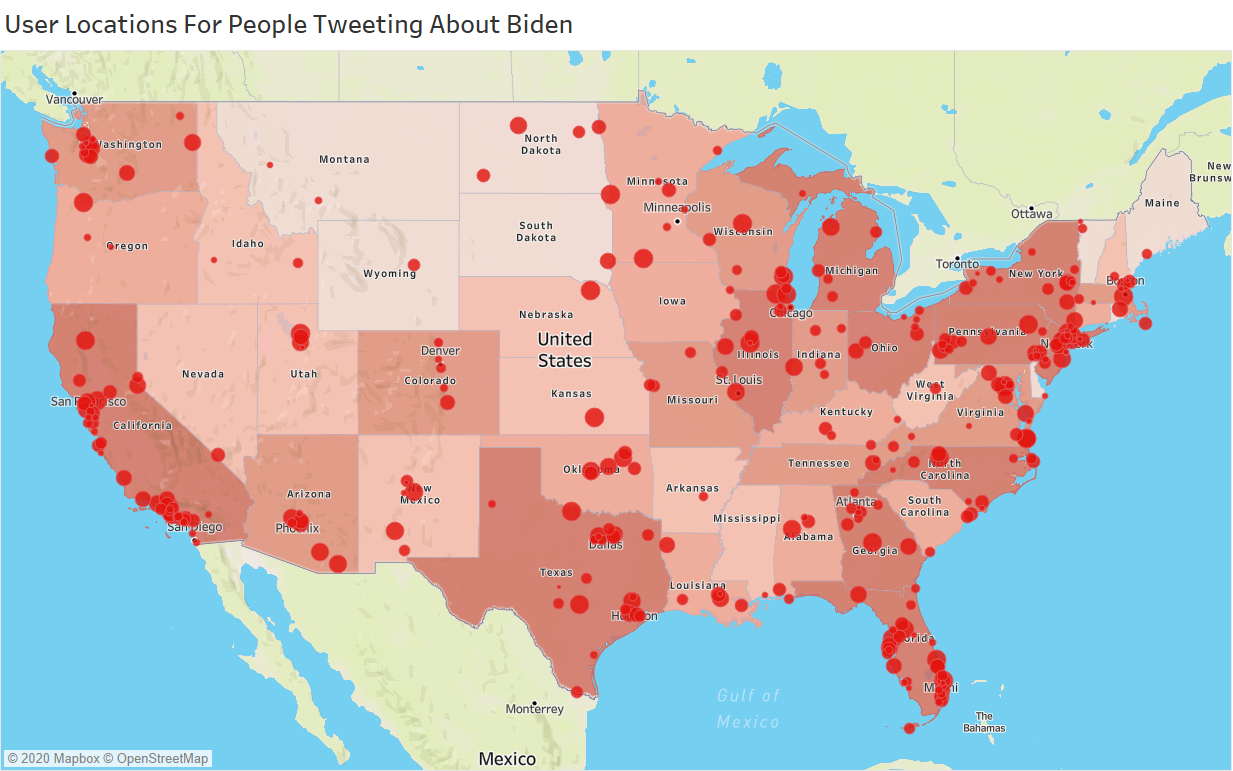##### This is a geomap. It shows the location of the users when they tweeted the respective tweets. We can observe that there’s a lot of density towards the eastern and south-east part of the United States which includes cities like Miami, Georgia, Virginia, North Carolina, Dallas, Chicago, Pennsylvania.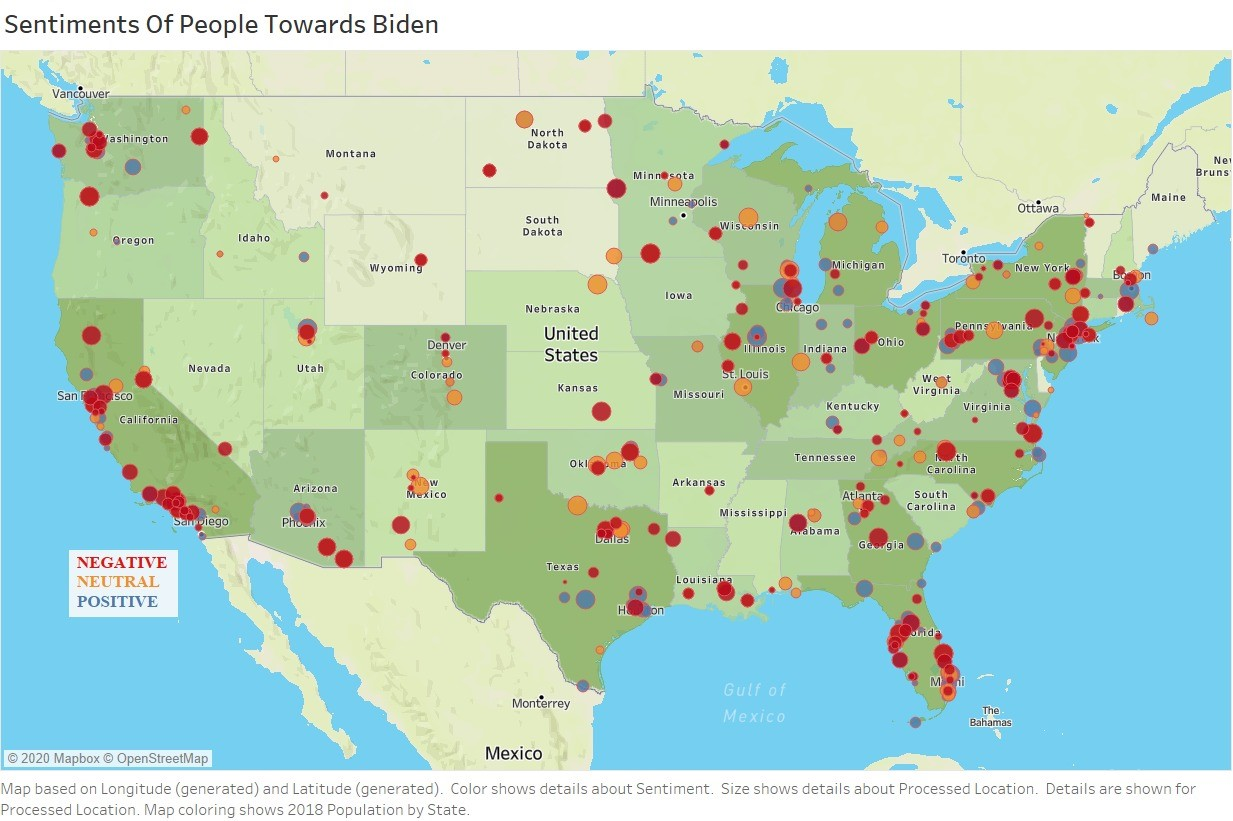##### This geomap shows the sentiment of people around the country.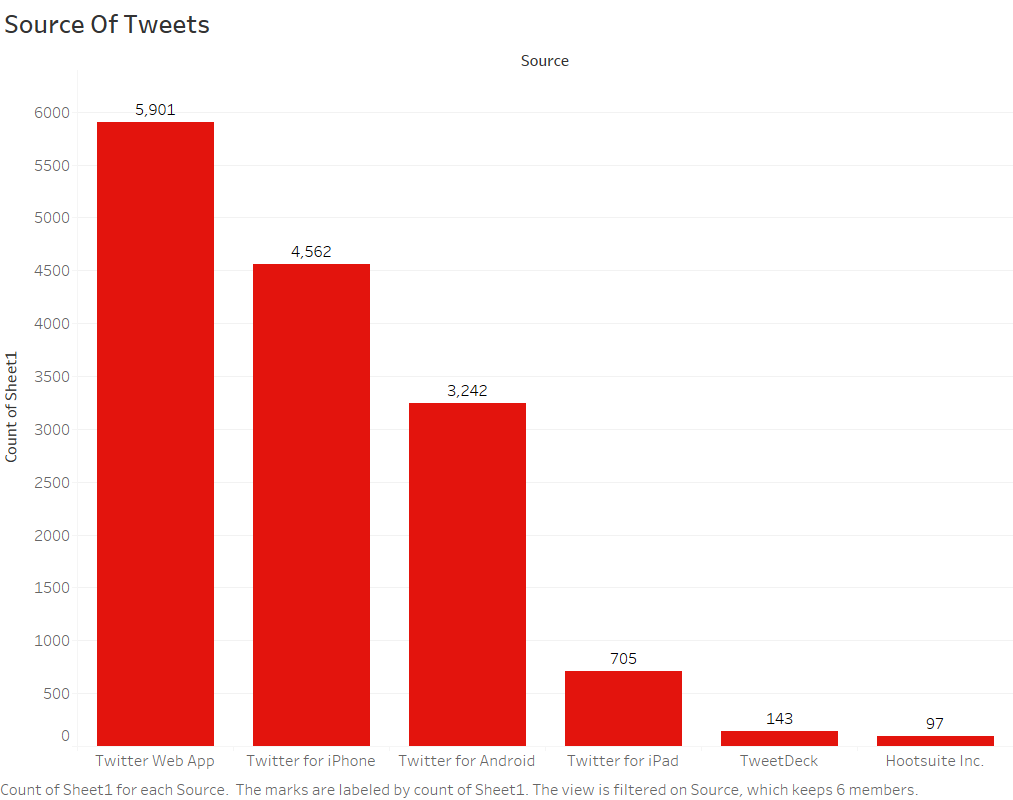##### This is a barplot with Source on X axis and Count on the Y axis. Source here refers to the medium used by the person to tweet, which can be Twitter Web App, Iphone, Android, ipad etc. Here we can see a major no. of user’s tweet using Web Application, followed by Iphone and the Ipad. The least used source is Hootsuite.com.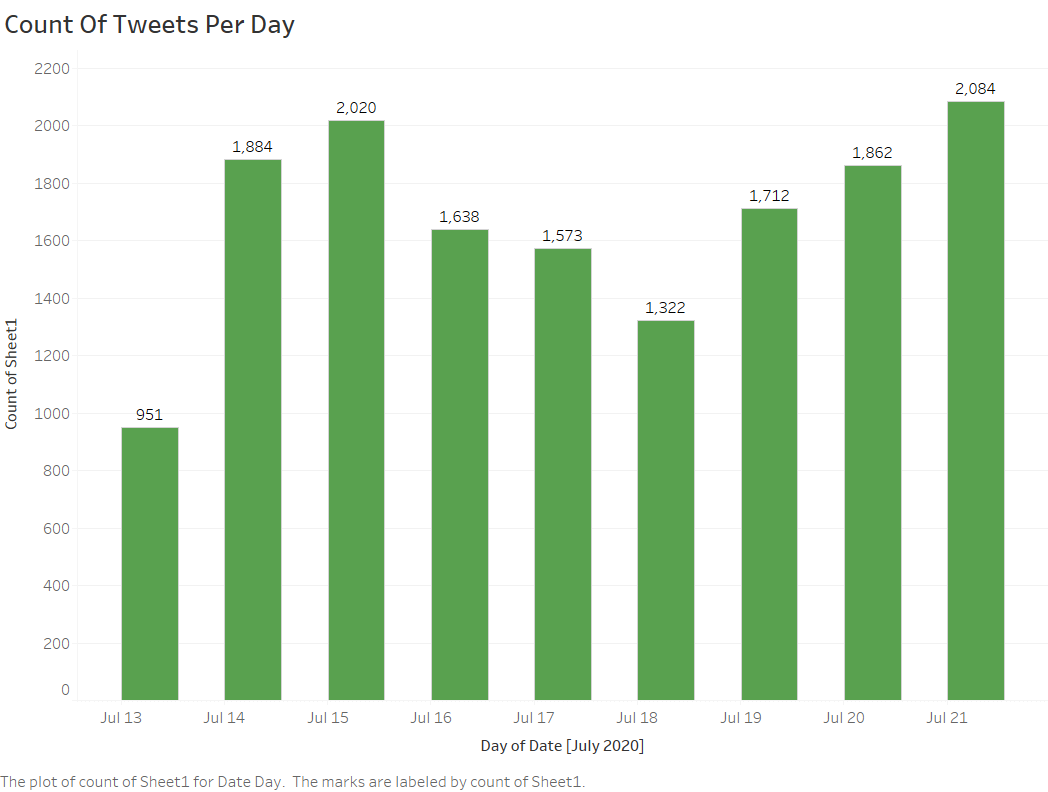##### The above barplot is depicting the counts of tweets per day for Biden in our time frame i.e. from July 13th to July 21st. The Y axis denotes the no. of tweets on the corresponding day on X axis. The maximum no. of tweets observed in the given timeframe is on July 21st.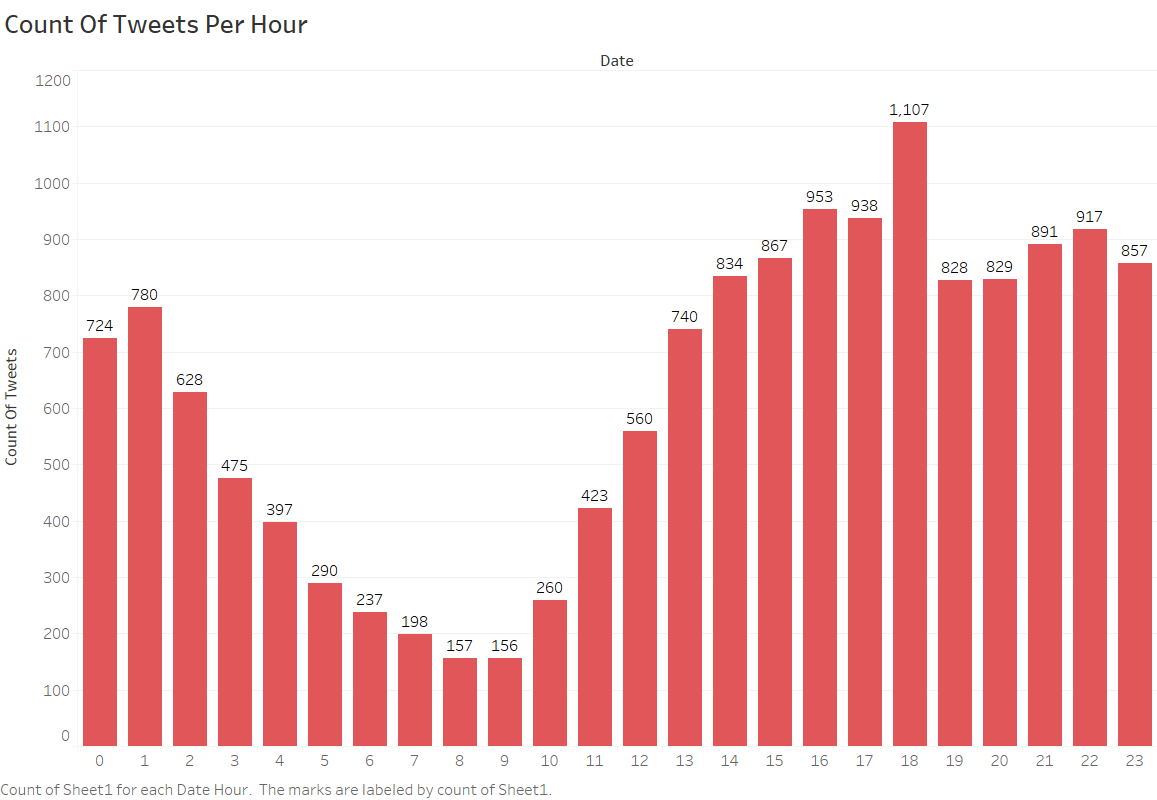##### The above graph is giving the no. of tweets hourwise on particular day. This shows the time of the day when users are most active, and that is around 17 : 00 hrs.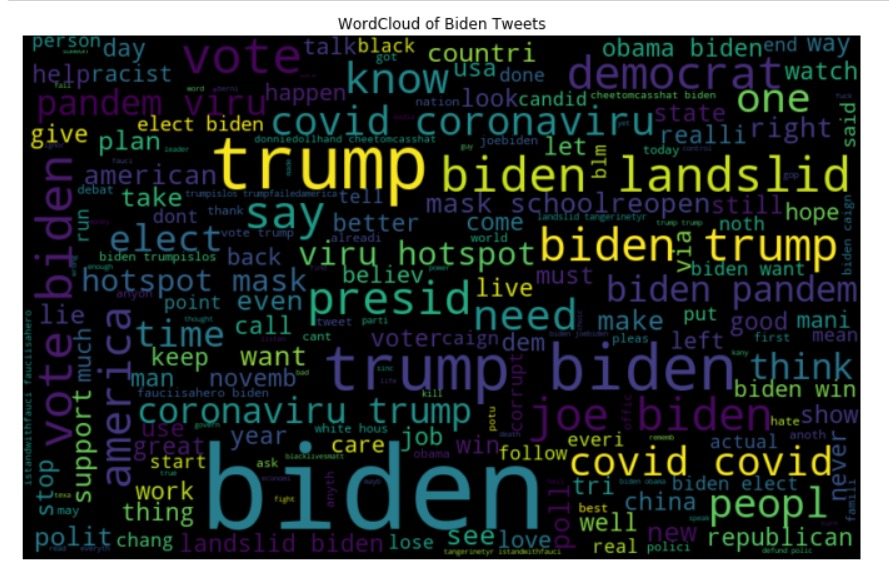### Donald Trump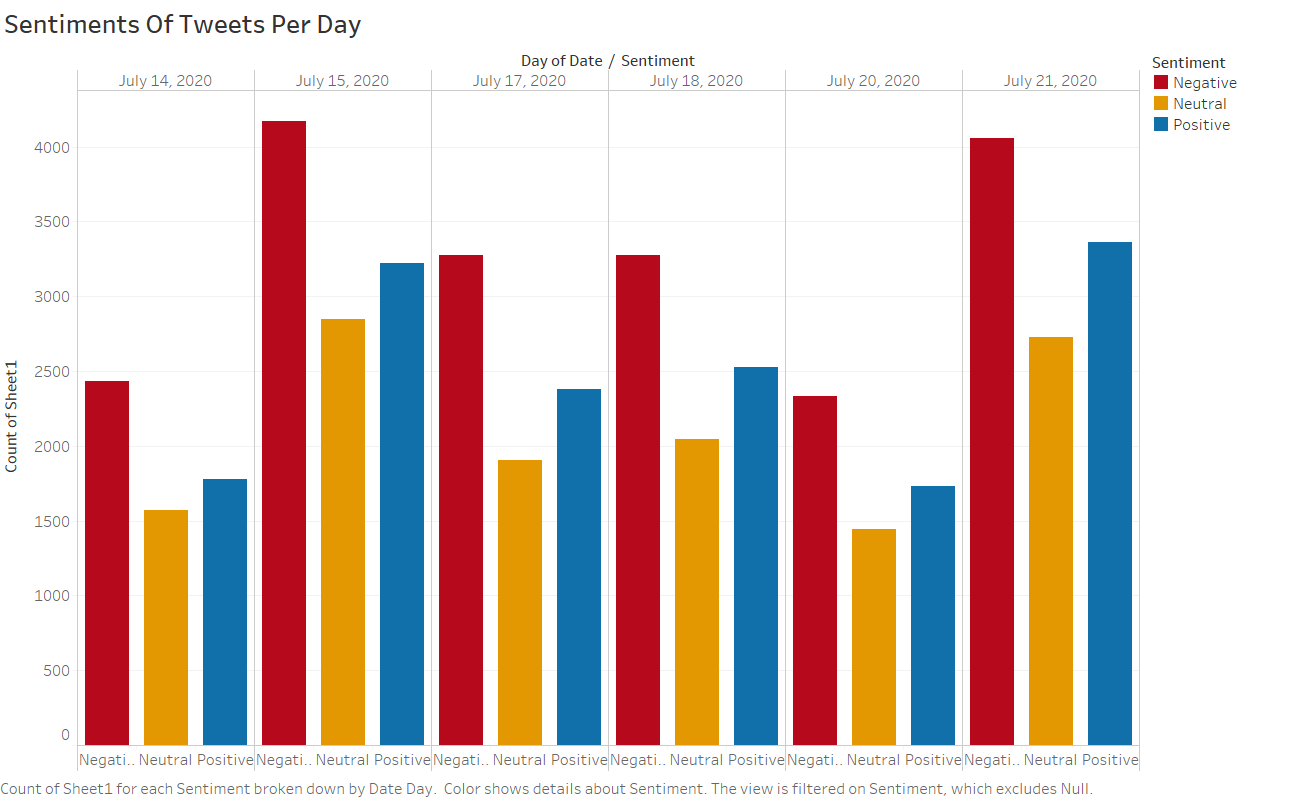##### In the above graph, we have the three broad sentiments on X axis and No. of tweets of that particular sentiment in our dataset, along with the dates of them being tweeted. A general observation here is that there is a majority of negative tweets on most of days. So, the general reaction towards Trump is unfavorable.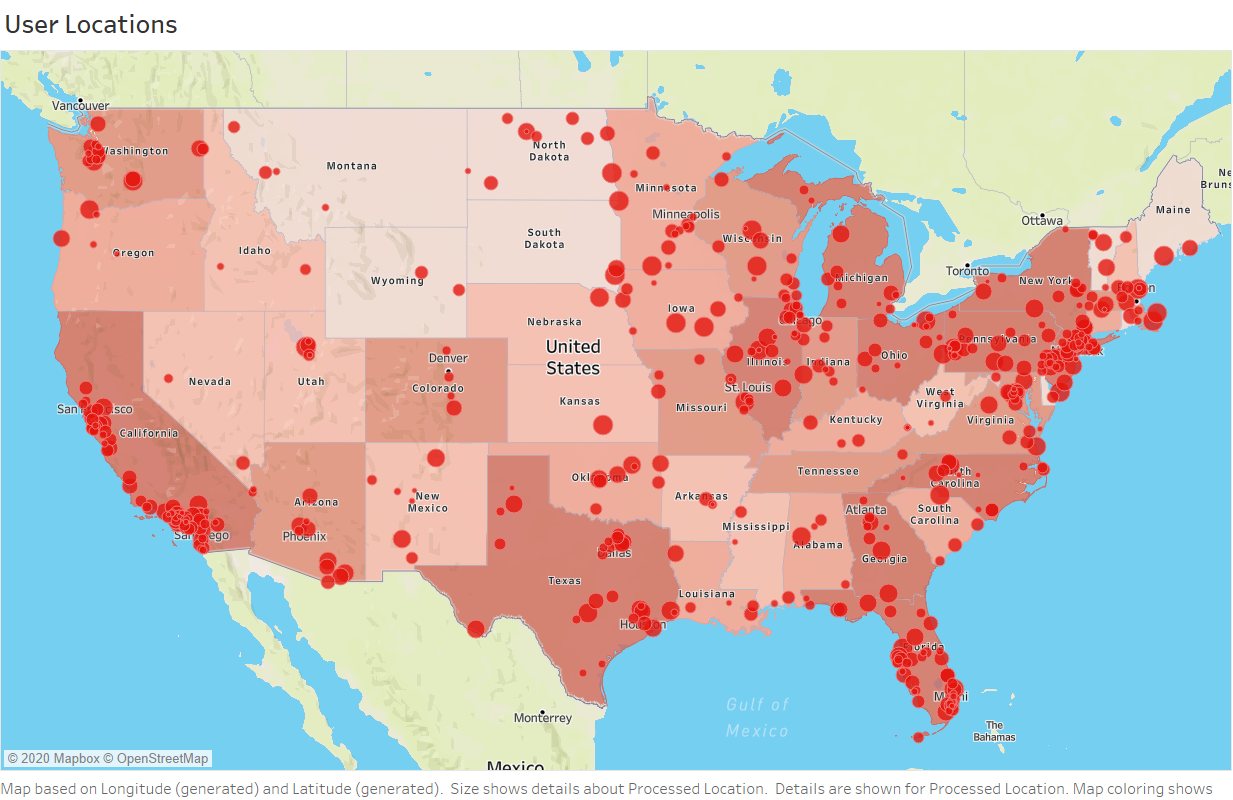##### This is a geomap. It shows the location of the users when they tweeted the respective tweets. We can observe that there’s a lot of density towards the eastern and south-east part of the United States which includes cities like New Jersey, Florida, New York, Massachusetts, Illinois, California.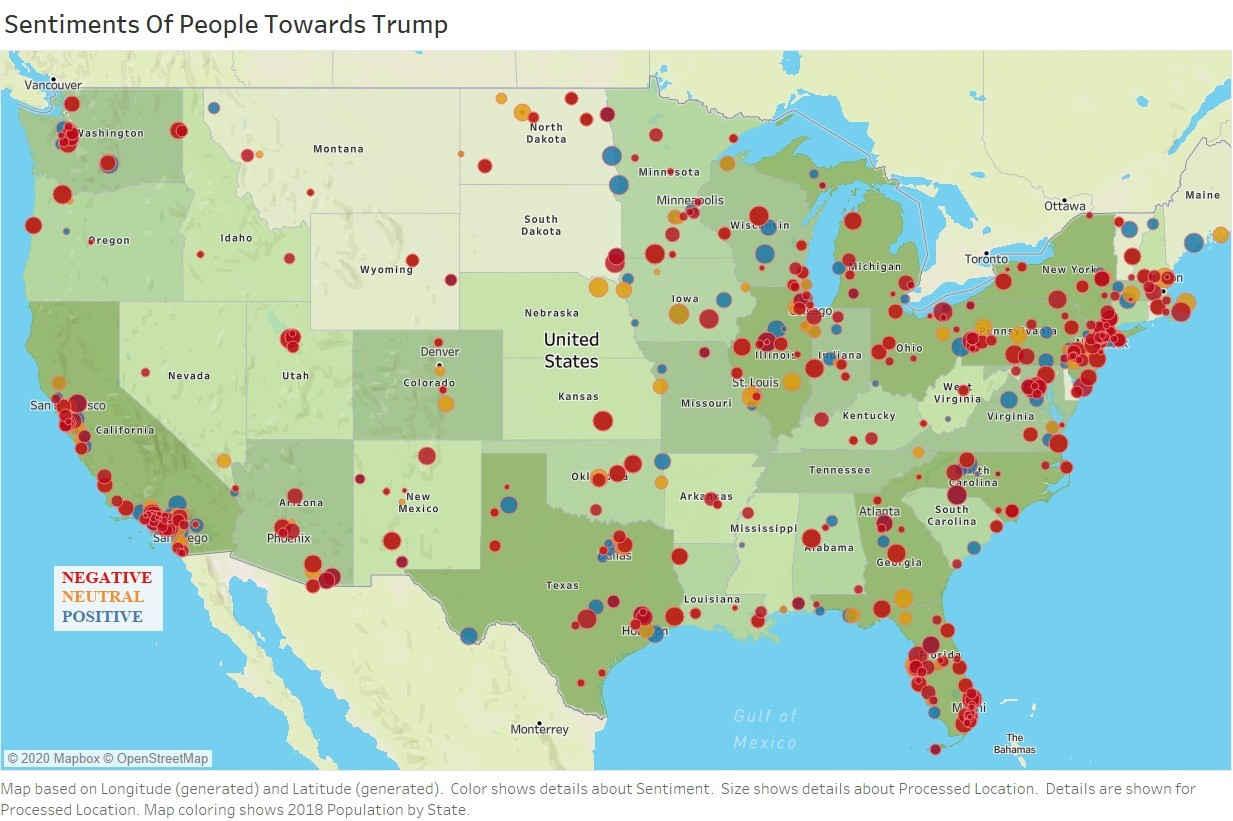##### This is a geomap. It shows the location of the users when they tweeted the respective tweets. We can observe that there’s a lot of red circles that denotes the negative response towards Trump.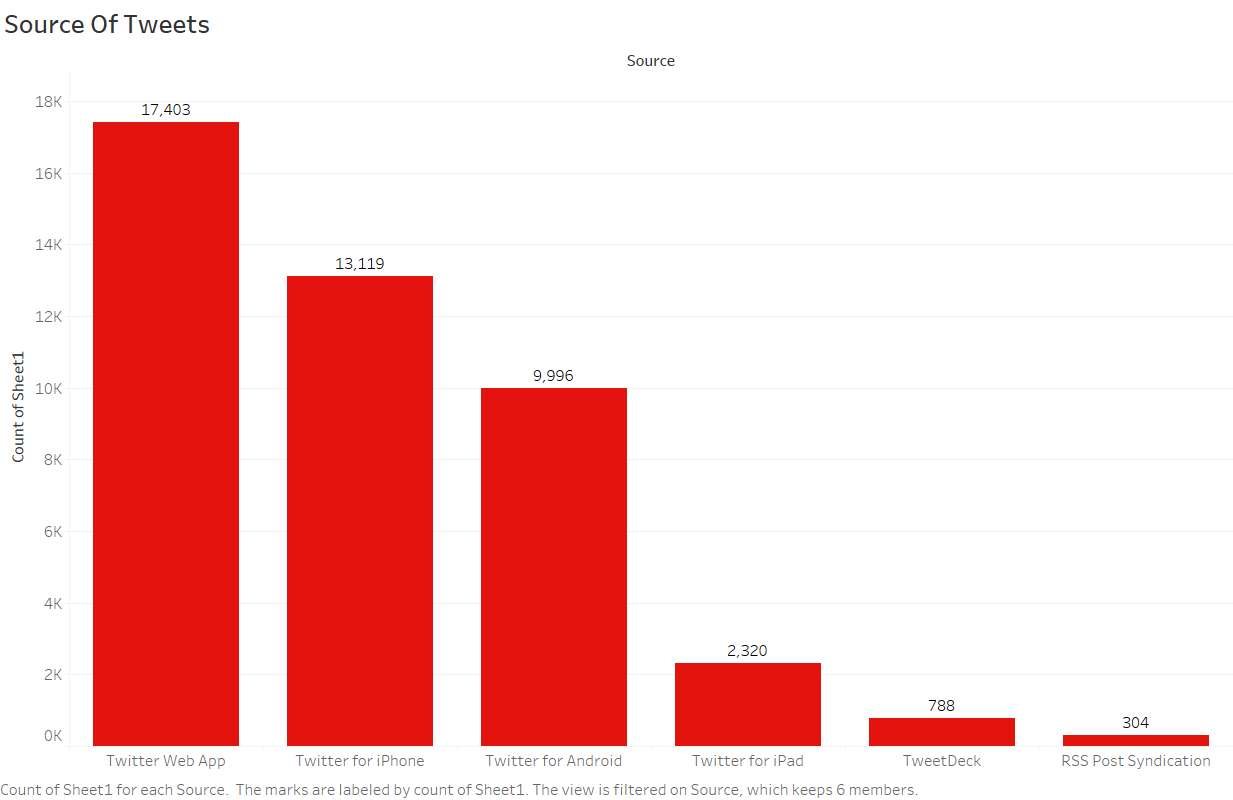##### This is a barplot with Source on X axis and Count on the Y axis. Source here refers to the medium used by the person to tweet, which can be Twitter Web App, Iphone, Android, ipad etc.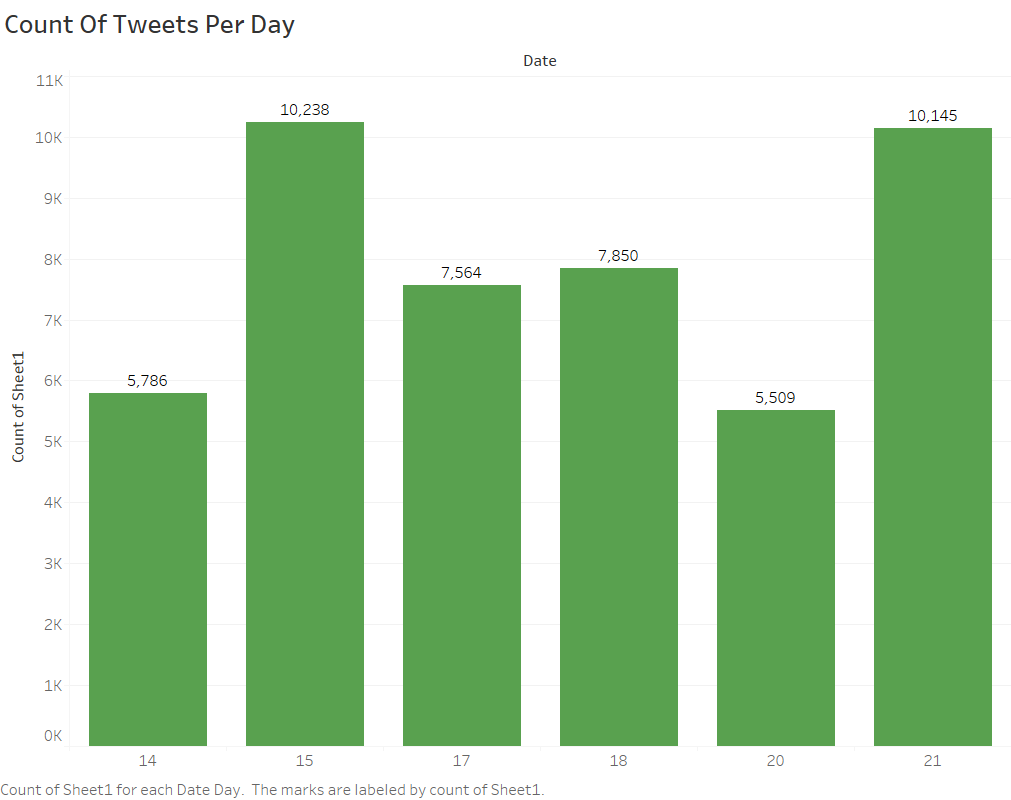##### The above barplot is depicting the counts of tweets per day for Biden in our time frame i.e. from July 14th to July 21st. The Y axis denotes the no. of tweets on the corresponding day on X axis. The maximum no. of tweets observed in the given timeframe is on July 15th.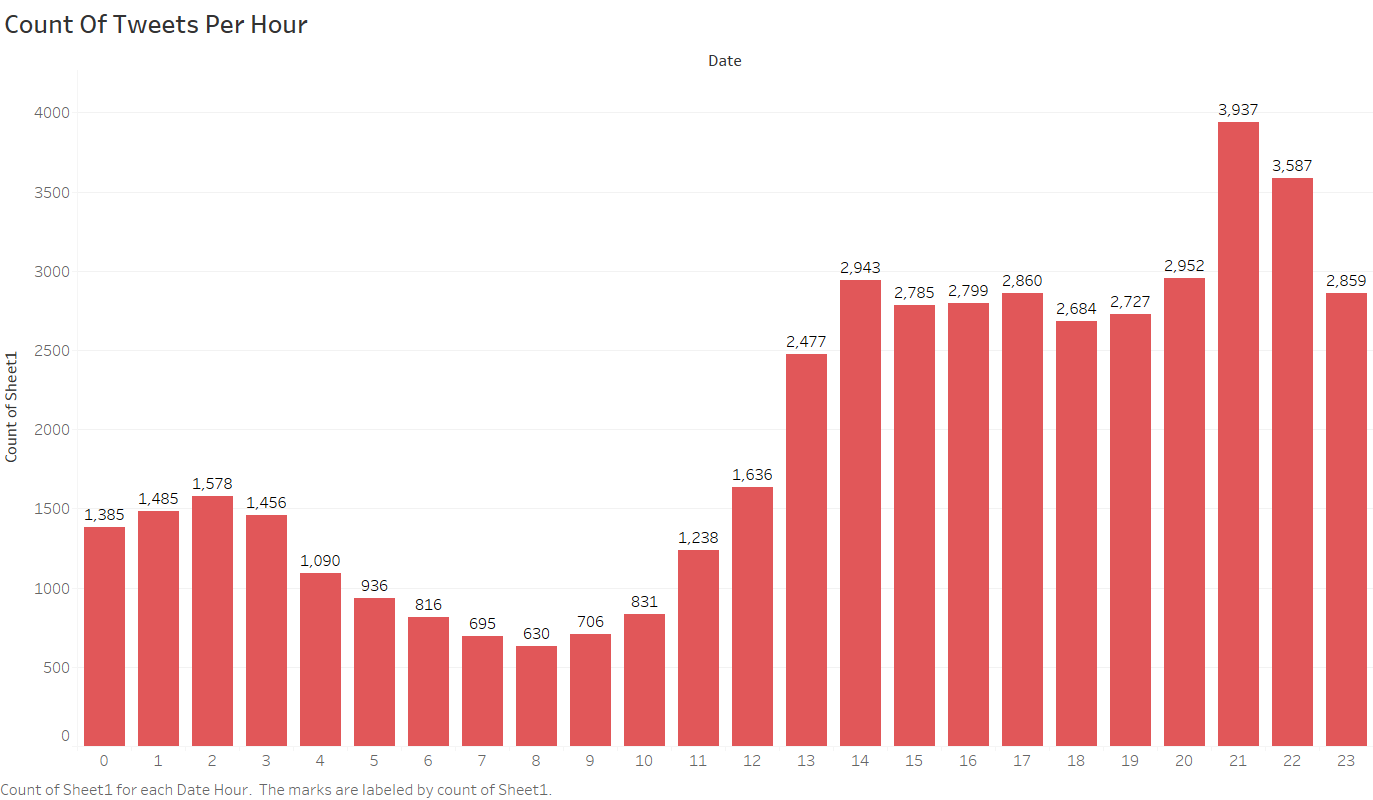##### The above graph is giving the no. of tweets hourwise on particular day. This shows the time of the day when users are most active, and that is around 21 : 00 hrs.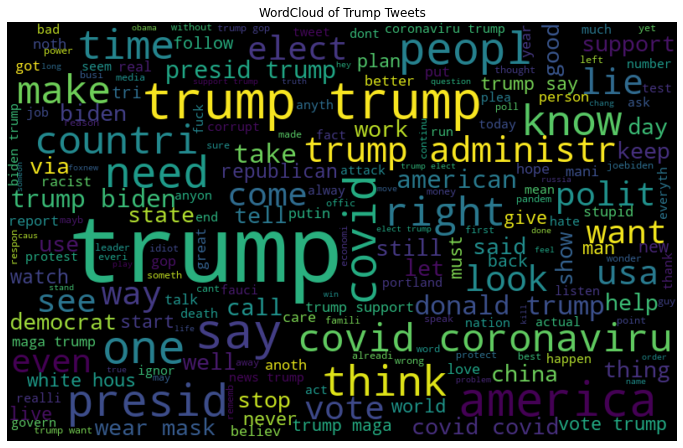### Kanye West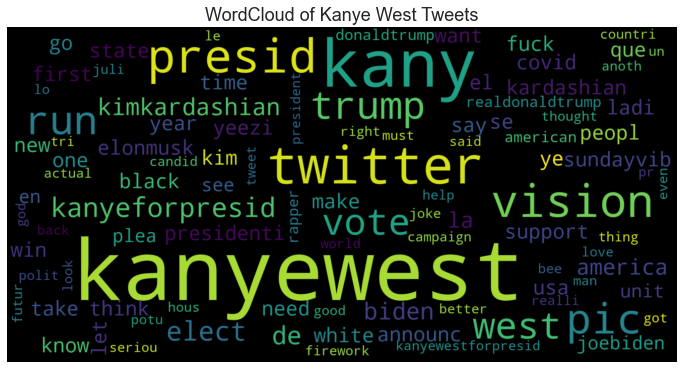##### WordCloud Of tweets for Kanye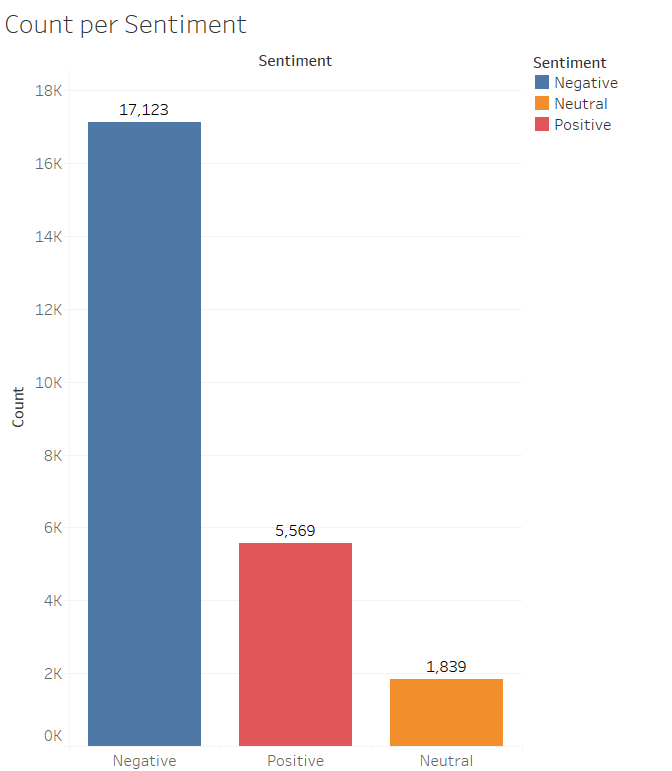##### As we can see from the graph, most of the population gave negative response towards Kanye.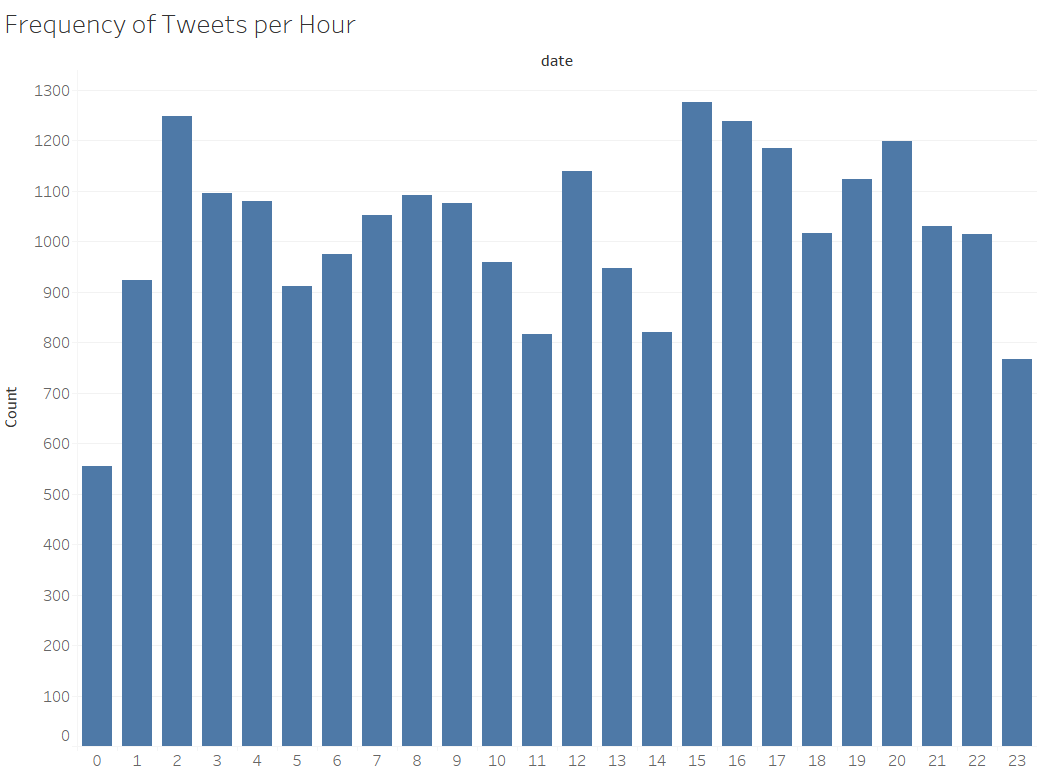##### The above graph is giving the no. of tweets hourwise on particular day. This shows the time of the day when users are most active, and that is around 15 : 00 hrs.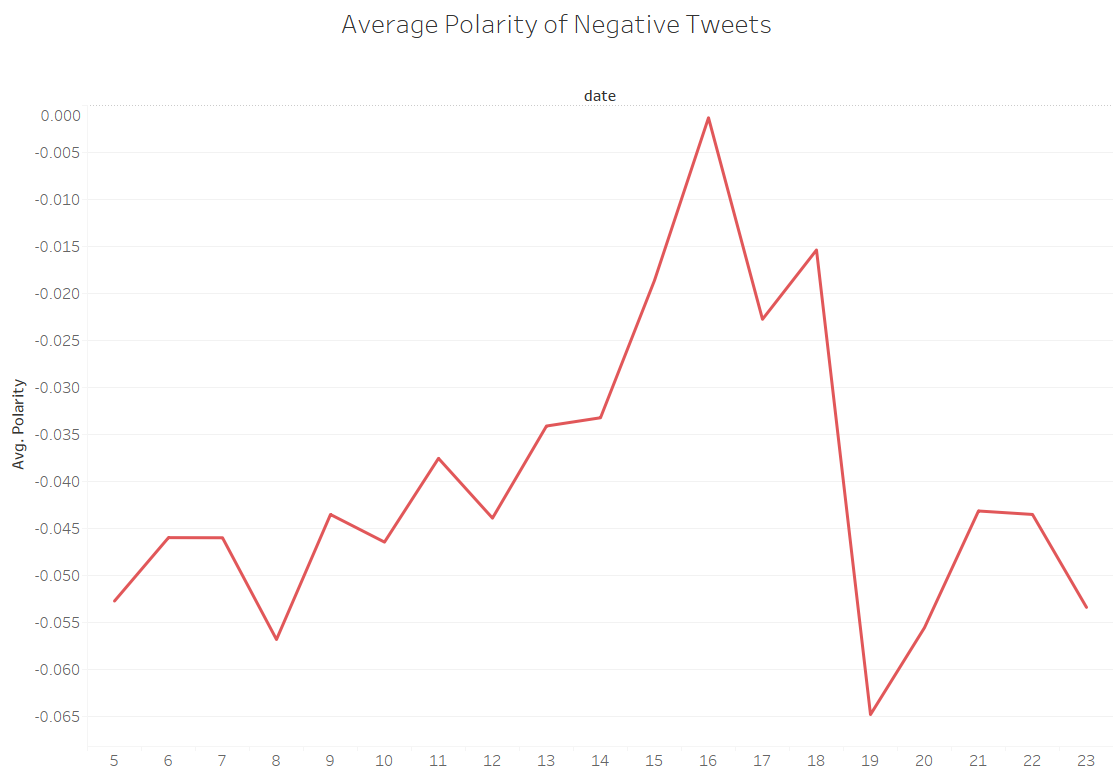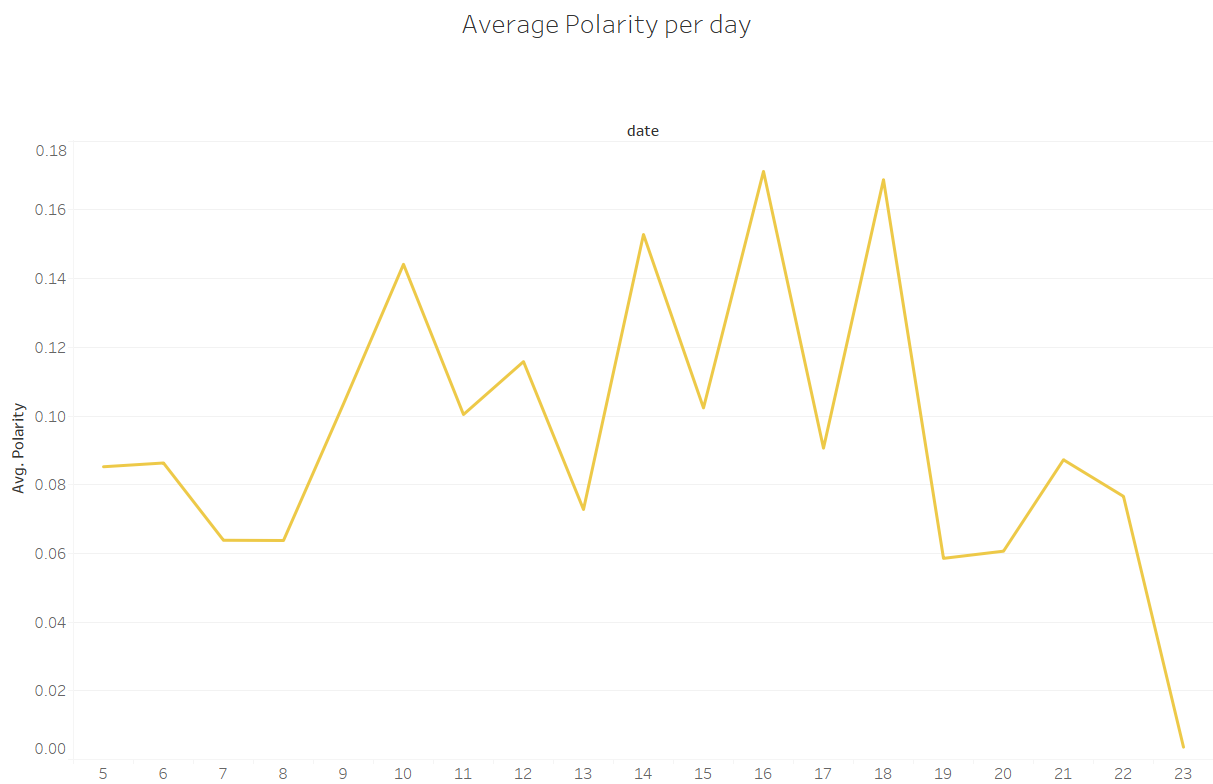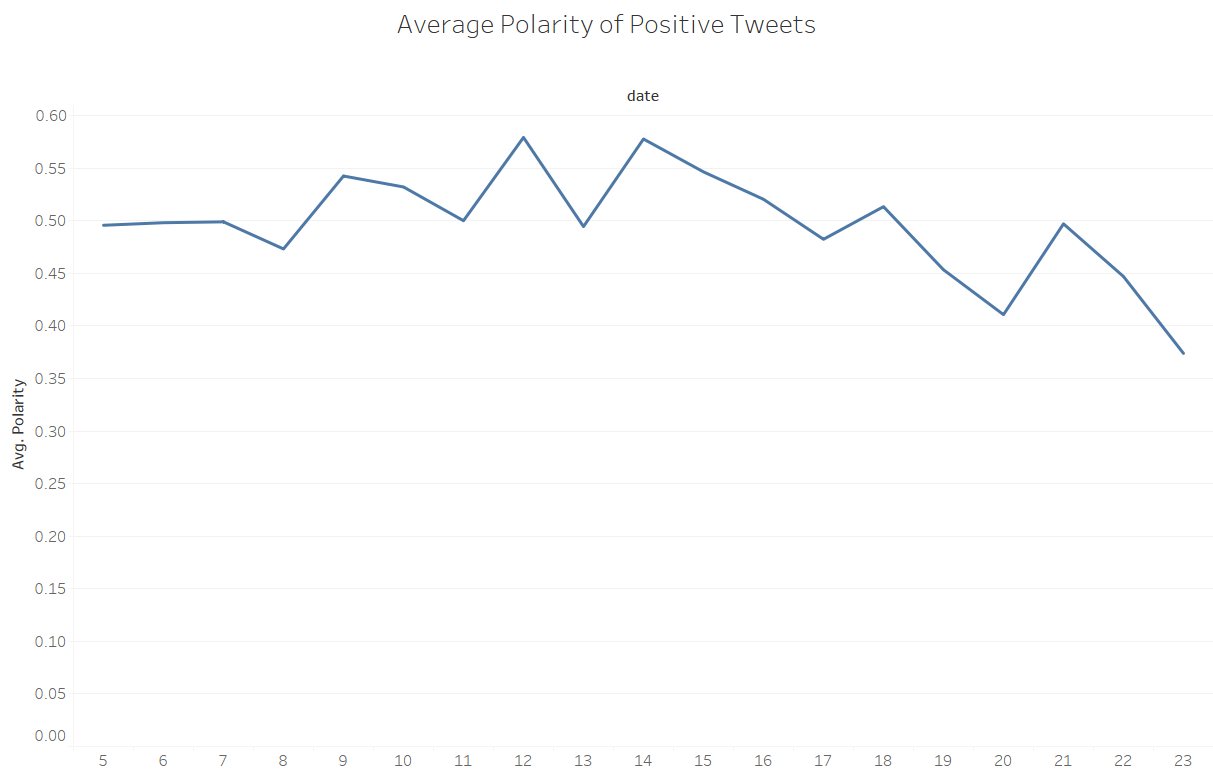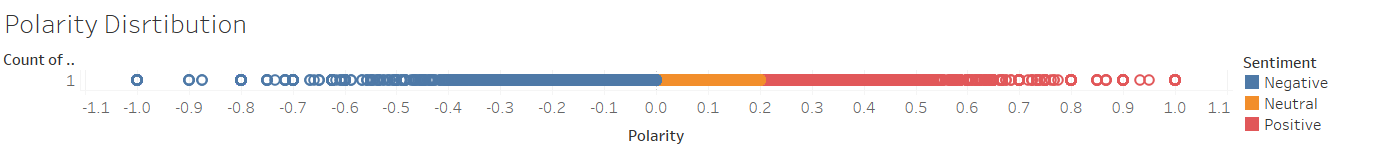Model Prediction

After completing the pre-processing, cleaning of the dataset and analysing the sentiment of the tweets, we can build the ML model. Before we can build the model, we need to transform the text data into the numerical features as we cannot directly feed the model the raw data. We can convert the text document into the numerical representation by vectorization.

There are 2 prominent methods:

1. Bag of Words:
2. TfIDF Vectorizer:

Bag of Words: It is a representation of text that describes the occurrence of words within a document. It involves : A. Vocabulary of known words. B. A measure of the presence of known words. It is called a ‘bag’ of words, because any information about the order or structure of words in the document is discarded. The model is only concerned with whether known words occur in the document, not where in the document. CountVectorizer package is used when implementing the Bag of Words model.

Problem with Bag of Words:

CountVectorizer simply gives equal weight to each of the word present in the document. If we have two different documents talking about the same topic but with different lengths, we observe that the average count values in the longer document will be higher than the shorter document. Hence we can avoid this problem by using the TfIDF Vectortizer- it evaluated the frequency of the words than the occurrence of the same word in the document, giving the importance of the words to that particular document.

TfIDF Vectorizer: TfiDF Vectorizer is a method to transforms text to feature vectors used to evaluate how important a word is to a document in a collection or corpus.

It is termed as the Term Frequency Inverse Document Frequency:

``````1.Term Frequency: The number of times the particular word appears in a single document.
2.Inverse Document Frequency: The log ratio of the total number of documents divided by the total documents where that particular word appears.
``````

Hence the TfiDf can be calculated by multiplying the two factors:

``````TfiDF = Tf * iDf
``````

Model Selection: We will be using the Naive Bayes, Random Forest, Decision Tree Classifier. We will observe the model behaviour in terms of its accuracy. Naïves Bayes Classifier: A Naive Bayes Classifier is a supervised machine-learning algorithm that uses the Bayes’ Theorem, which assumes that features are statistically independent. Bayes’ Theorem, which is based on conditional probability or in simple terms, the likelihood that an event (A) will happen given that another event (B) has already happened. Essentially, the theorem allows a hypothesis to be updated each time new evidence is introduced. The equation below expresses Bayes’ Theorem in the language of probability

• “P” is the symbol to denote probability.

• P(A | B) = The probability of event A (hypothesis) occurring given that B (evidence) has occurred.

• P(B | A) = The probability of the event B (evidence) occurring given that A (hypothesis) has occurred.

• P(A) = The probability of event B (hypothesis) occurring.

• P(B) = The probability of event A (evidence) occurring.

Random Forest:

A Random Forest is an ensemble technique capable of performing both regression and classification tasks with the use of multiple decision trees and a technique called Bootstrap and Aggregation, commonly known as bagging. The basic idea behind this is to combine multiple decision trees in determining the final output rather than relying on individual decision trees.

Decision Tree :

Decision tree is a supervised algorithm used to solve both regression and classification problems. It uses the tree representation to solve the problem in which each leaf node corresponds to a class label and attributes are represented on the internal node of the tree.

The approach used here is splitting the dataset into training and testing dataset- using the training set to train the model and comparing the testing set with the predicted values from the model.

Model Evaluation:

There are different approaches used for the model evaluation. Since our problem falls under classification we need to identify which class defines positive result and which one negative. In our case, we assume that predicting ‘positive’ class is a positive result. If our model is predicting input text as ‘positive’ and it really has ‘positive’ label in test data, then it is called True positive. If our model predicted an input text as ‘positive’ though it is ‘negative’ in reality, it is called False Positive. While checking the model performance, we should always consider test data. Using training data will not make much sense as our model will be biased to give the actual prediction.

• Confusion Matrix: This matrix helps you to understand the types of errors made by our classifier.

• Accuracy: It measures the percentage of correct predictions .i.e the number of correct predictions made by our model divided by the total number of predictions.

• Recall: Recall measures the ability of the classifier to find all the positive data points.

• Precision: It measures the proportion of correct positive predictions out of all positive predictions made by our model.

• F1 score: F1 Score is the weighted average of Precision and Recall. Therefore, this score takes both false positives and false negatives into account.

### Result:

As mentioned above,people are most affirmative towards Joe Bidden. Hence our model predicts that Joe Bidden will win the elections.

Deep Learning Model

After pre-processing of the dataset, and obtaining a new column in the dataset, which carries the ‘Sentiment’ pertaining to the respective tweet, we come to the part where we use concepts of NLP to build and train a model that predicts the sentiment of the tweets fed into the model. Setting the Hyper Parameters and constants (Only the best parameters are displayed below):

• Embedding Dimension = 150 • Maximum Length of input for Embedding = 200 • Truncating Type = “post” • Padding Type = “post” • Out of Vocabulary token = “” • Training Portion = 0.8

Using the training portion, the data is splitted in training and validation sets : 80% Training, 20% Validation. The target set is One-Hot-Encoded as [‘Negative’, ‘Neutral’, ‘Positive’] Tokenization and Padding of the sentences is performed using tokenizer from tf.keras.preprocessing.text and pad_sequences from tf.keras.preprocessing.sequences. Defining the Model: Using Sequential, the layers in the model are as follows:

• Embedding : Vocabulary Size = 15995 , Embedding Dimension = 150, Input Length = 200 • Bidirectional LSTM : Nodes = 256 • Dropout : 0.5 • BatchNormalization • Dropout : 0.5 • Flatten • Dense : Nodes = 64, activation = ‘relu’ • Dropout : 0.5 • Dense : Nodes = 3, activation = ‘softmax

Model is compiled using model.compile():

• Optimizer = Adam (learning rate = 9e-4) • Loss = ‘categorical_crossentropy’ • Metrics = Accuracy Model is fit on the dataset using model.fit() : Epochs = 7

All the metrics observed during the model training are displayed on one plot :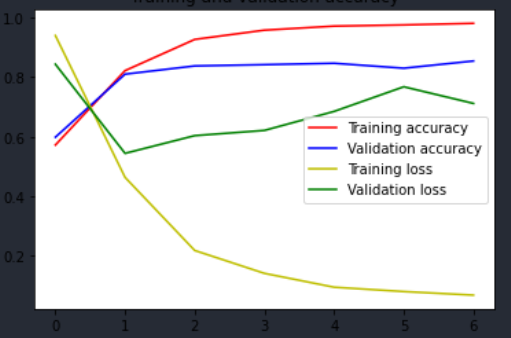##### Now some tweets pertaining to Joe Biden are passed through the model, and prediction of sentiment is displayed.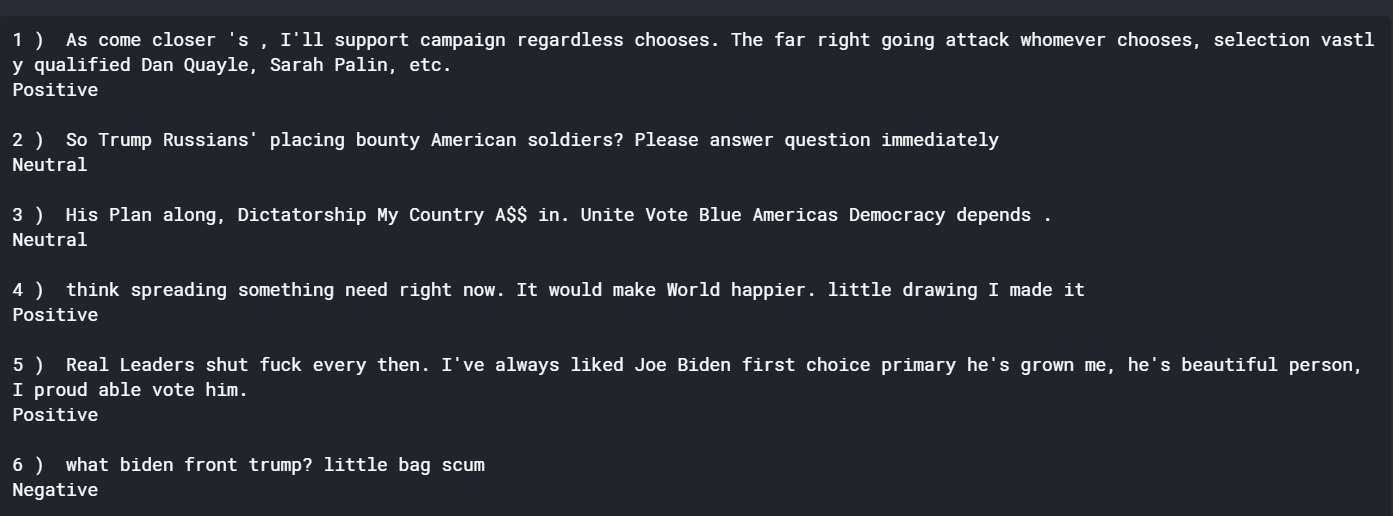## ContributorsMade with ❤️ by DS Community SRM

 Shubhangi SoniRohan MathurTejas ChintalaBhavya ChhabraSampada BarejaGet A Weekly Email With Trending Projects For These Topics
No Spam. Unsubscribe easily at any time.
Jupyter Notebook (154,575
Machine Learning (37,158
Deep Learning (36,543
Nlp (14,783
Regular Expression (8,798
Tweets (8,386
Sentiment (6,179
Sentiment Analysis (3,259
Preprocessing (2,278
Nltk (1,979
Classification Model (196
Tweepy Api (48
Tableau Desktop (37